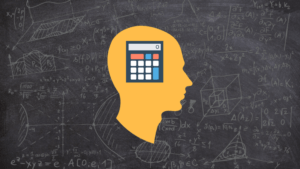[s2If current_user_can(access_s2member_ccap_mentalmath)]Mental Math: Tricks To Be A Human Calculator
The Only System To Do Speed Mental Math In Your Head Faster Than A Calculator Making Fast Mental Math Your New Super Power.
 Module 1 Welcome Welcome to Ofpad Mental Math System Unit 1 What Is The Ofpad Mental Math System? Module 2 DS Method Of Checking Quickly check the answer of any math problem. Unit 1 DS Method of Checking Calculation Unit 2 The Digit Sum Parlour Trick Module 3 Introduction To Mental Math Introduction to course structure by learning how to multiply by 11. Unit 1 Introduction to Mental Math Unit 2 Quiz - Introduction to Mental Math Module 4 LR Addition & Subtraction Variations of LR method applied to addition and subtraction. Unit 1 The Secret Of Mental Math Unit 2 LR Method for Addition & Subtraction Unit 3 To Round Up or Not Round Up Unit 4 Quiz - Addition Unit 5 Quiz - Subtraction Unit 6 Getting Free Drinks Guessing Barcodes Module 5 LR Multiplication Variations of LR method applied to multiplication. Unit 1 LR Multiplication Method Unit 2 Quiz - LR Multiplication (One Digit Multiplier) Unit 3 Quiz - LR Multiplication (Two Digit Multiplier) Module 6 Squaring You will learn how to square numbers quickly Unit 1 Squaring Unit 2 Quiz - Squaring Module 7 Advanced Multiplication Advanced Multiplication Method When LR Method is Difficult. Unit 1 The Bridge Method of Multiplication Unit 2 Vitruvian Man Method for Multiplication Unit 3 Quiz - Advanced Mental Multiplication Module 8 UT Method Another technique to multiply really large and complex numbers quickly and efficiently. Unit 1 UT Multiplication Unit 2 Quiz - UT Multiplication Unit 3 Using The Different Multiplication Techniques Module 9 FP Division Divide numbers quickly in your head. This will take practice to truly master. Unit 1 FP Division Unit 2 Quiz - FP Division Module 10 Bonus Training Bonus weekly practice to take your mental math skills to the next level. Unlocks every week. Unit 1 Bonus

[else]

Watch This Shocking Presentation Which Reveals A Simple Secret To Become a Human Calculator